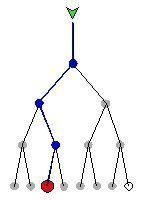Definition

binary tree

A binary tree is a method of placing and locating files (called records or keys) in a database, especially when all the data is known to be in random access memory (RAM). The algorithm finds data by repeatedly dividing the number of ultimately accessible records in half until only one remains.

In a tree, records are stored in locations called leaves. This name derives from the fact that records always exist at end points; there is nothing beyond them. Branch points are called nodes. The order of a tree is the number of branches (called children) per node. In a binary tree, there are always two children per node, so the order is 2. The number of leaves in a binary tree is always a power of 2. The number of access operations required to reach the desired record is called the depth of the tree. The image to the left shows a binary tree for locating a particular record among seven records in a set of eight leaves. The depth of this tree is 4.In a practical tree, there can be thousands, millions, or billions of records. Not all leaves necessarily contain a record, but more than half do. A leaf that does not contain a record is called a null. In the example shown here, the eighth leaf is a null, indicated by an open circle.

Binary trees are used when all the data is in random-access memory (RAM). The search algorithm is simple, but it does not minimize the number of database accesses required to reach a desired record. When the entire tree is contained in RAM, which is a fast-read, fast-write medium, the number of required accesses is of little concern. But when some or all of the data is on disk, which is slow-read, slow-write, it is advantageous to minimize the number of accesses (the tree depth). Alternative algorithms such as the B-tree accomplish this.

Also see binary search and tree structure. Compare B-tree, M-tree, splay tree, and X-tree.

This was last updated in September 2005

Close